# RotatE: Knowledge Grpah Embedding by Relational Rotation Complex Space

## Basic Idea

1. 在许多任务中的成功推断都严重依赖于关系模式.
2. 现有的Knowledge Embedding Model都不能对所有关系同时建模.

## RotatE

### Modeling and Inferring Relation Patterns

1. 对称关系和反对称关系:

对称关系: 如果$x$ 能通过变换$r$ 得出$y$, 那么$y$ 必然能通过同样的$r$ 得出$x$.

反对称关系: 若$x$ 能通过$r$ 找到$y$, 那么$y$ 必然不能通过同样的$r$ 找到$x$, 或者说$y$ 对$x$ 必然不存在关系$r$.

即:

\begin{aligned} r(x, y) &\Rightarrow r(y, x) \\ r(x, y) &\Rightarrow \neg r(y, x) \end{aligned}

1. 互逆关系:

如果$r_1$ 和$r_2$ 互为逆关系, $x$ 必然能通过$r_1$ 得到$y$, 同时$y$ 也能通过$r_1$ 的逆变换$r_2$ 得到$x$, 即:

$$r_{2}(x, y) \Rightarrow r_{1}(y, x)$$

1. 组合关系:

如果$r_3$ 是由变换$r_1$ 和变换$r_2$ 组成的, 那么它们之间存在递进关系, 即:

$$r_{2}(\mathrm{x}, \mathrm{y}) \wedge \mathrm{r}_{3}(\mathrm{y}, \mathrm{z}) \Rightarrow \mathrm{r}_{1}(\mathrm{x}, \mathrm{z})$$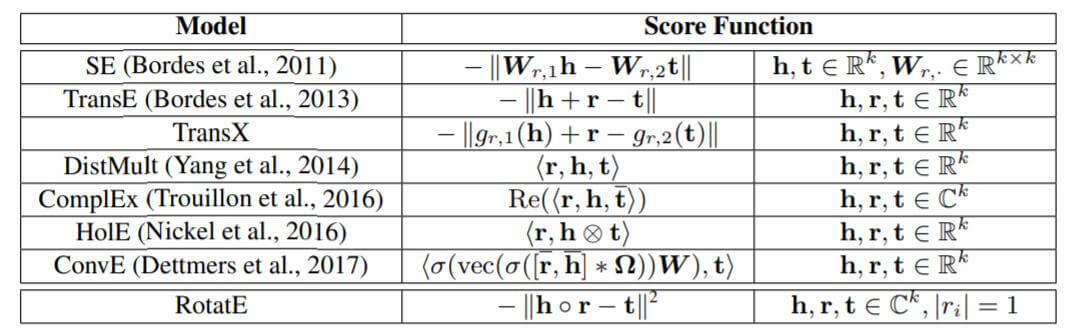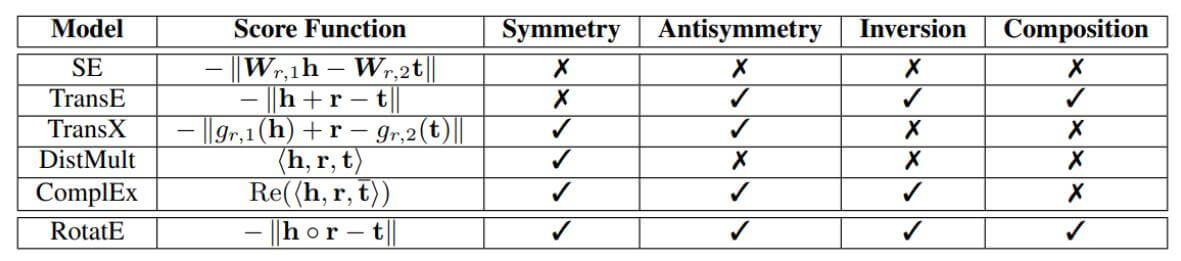### Modeling Relations as Rotations in Complex Vector Space

#### Euler’s Inspired

RotatE的灵感来源于欧拉公式:
$$e^{i \theta}=\cos \theta+i \sin \theta$$

1. 对称关系: 将$x$ 旋转$\theta=\pi$ 得到$y$, 在$y$ 处再次旋转$\theta=\pi$ 回到$x$.
2. 互逆关系: 将$x$ 旋转$\theta_1$ 得到$y$, 在$y$ 处旋转$\theta_2=-\theta_1$, 即反方向旋转$\theta_1$, 可以回到$x$.
3. 组合关系: 将$x$ 先旋转$\theta_1$, 记为$y$, 再旋转$\theta_2$ 记为$z$. 该过程等价于从$x$ 处旋转$\theta_3=\theta_1+\theta_2$, 直接得到$z$.

$$\mathbf{t}=\mathbf{h} \circ \mathbf{r}$$

$$d_{r}(\mathbf{h}, \mathbf{t})=\lVert\mathbf{h} \circ \mathbf{r}-\mathbf{t}\rVert$$

#### Connection to TransE

TransE仅在一维数轴上做关系变换, 而将关系变换定义为旋转的RotatE可以在二维复平面上做关系变换: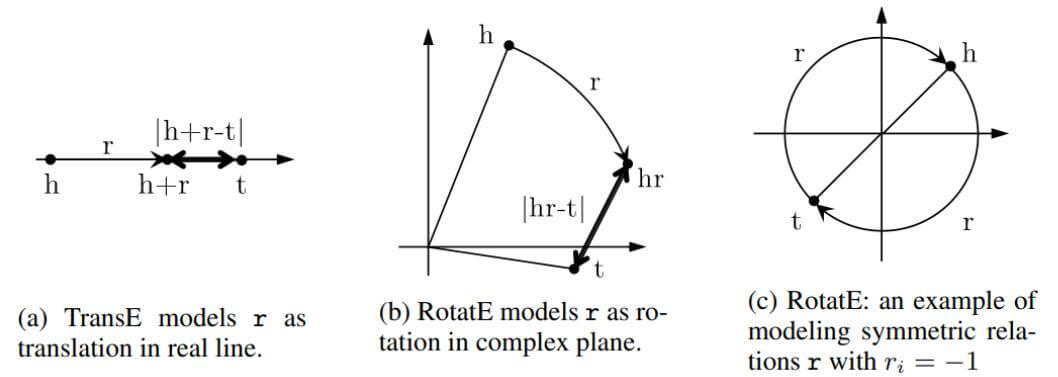### Optimization

#### Loss Function

RotatE仍然采用类似TransE的Hinge Loss, 并加上负采样:
$$L=-\log \sigma\left(\gamma-d_{r}(\mathbf{h}, \mathbf{t})\right)-\sum_{i=1}^{n} \frac{1}{k} \log \sigma\left(d_{r}\left(\mathbf{h}_{i}^{\prime}, \mathbf{t}_{i}^{\prime}\right)-\gamma\right)$$

#### Self - Adversarial Negative Sampling

$$p\left(h_{j}^{\prime}, r, t_{j}^{\prime} \mid\left\{\left(h_{i}, r_{i}, t_{i}\right)\right\}\right)=\frac{\exp \alpha f_{r}\left(\mathbf{h}_{j}^{\prime}, \mathbf{t}_{j}^{\prime}\right)}{\sum_{i} \exp \alpha f_{r}\left(\mathbf{h}_{i}^{\prime}, \mathbf{t}_{i}^{\prime}\right)}$$

$$L=-\log \sigma\left(\gamma-d_{r}(\mathbf{h}, \mathbf{t})\right)-\sum_{i=1}^{n} p\left(h_{i}^{\prime}, r, t_{i}^{\prime}\right) \log \sigma\left(d_{r}\left(\mathbf{h}_{i}^{\prime}, \mathbf{t}_{i}^{\prime}\right)-\gamma\right)$$

## Experiments

### Main Results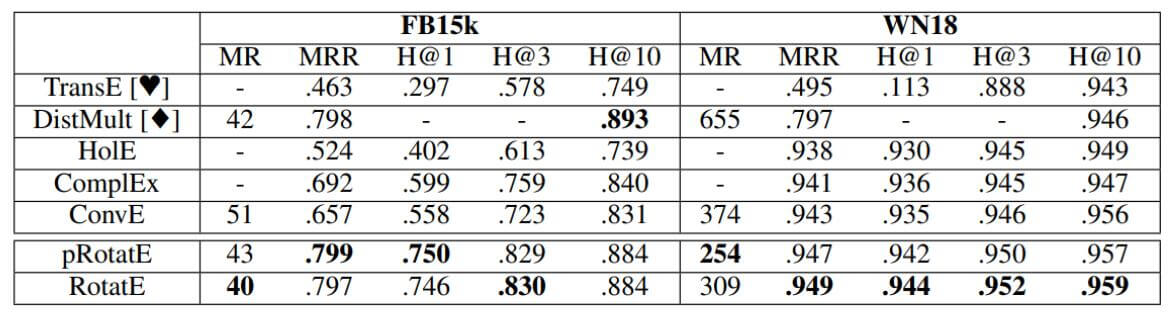RotatE表现出很强大的性能, 和变体差不多.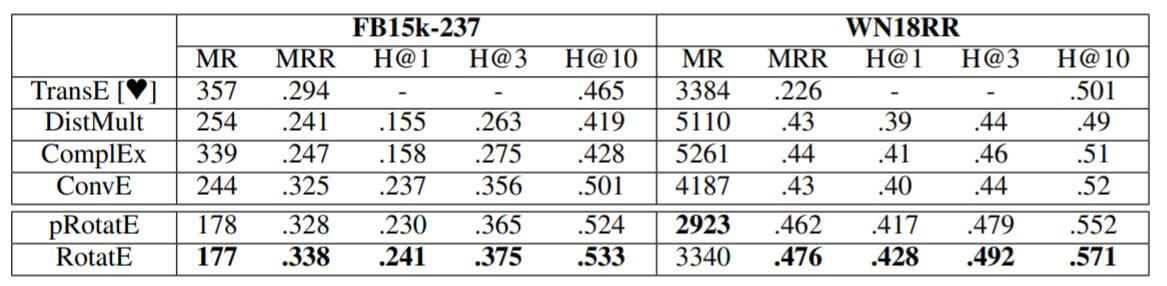RotatE比ConvE的结果要好一些. 但对于MR来说, RotatE的结果要好得多, 说明ConvE对于某些关系学习到的特征是模糊的.

### Relation Pattern Experiments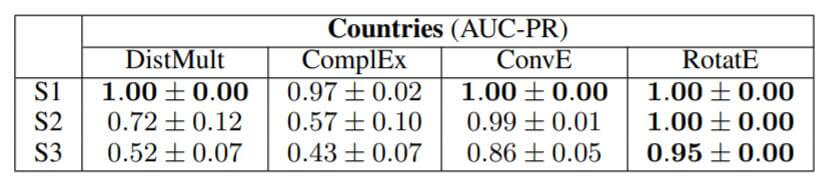WN18上的各类关系所对应的$r$ 值如下: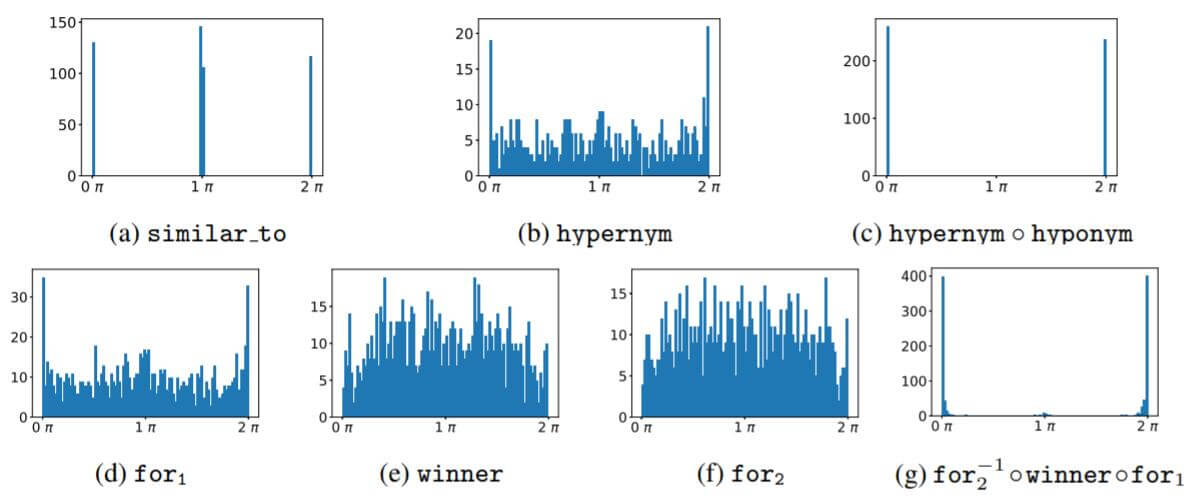similar_to是明显的对称关系, hypernym, hyponym是一对明显的逆关系, 二者的组合刚好是什么也不做, for1, for2, winner三者的组合也被学习到了. RotatE很好的掌握了这些关系的变换方式.

### Negative Sampling Techniques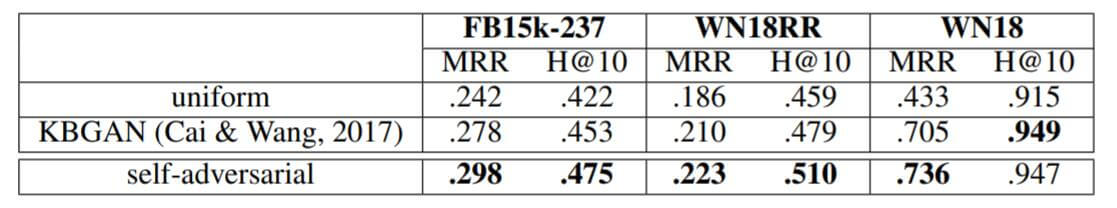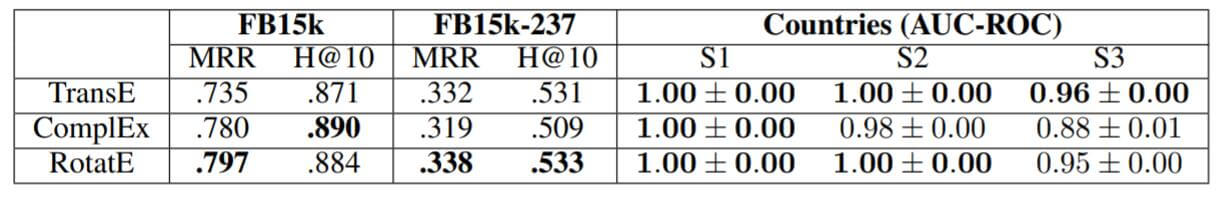RotatE仍然是最优秀的方法. 但TransE比RotatE在S3上稍好一些, 作者解释为Countries不包含TransE最不擅长的对称关系, 在这个数据集上显示出TransE的强大.

### Results by Relation Category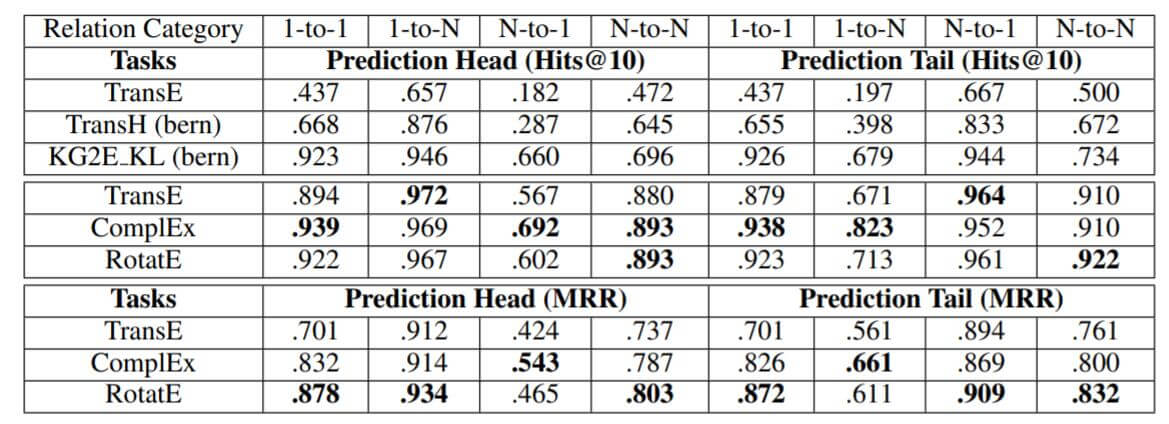## Summary

RotatE将关系变换定义为旋转, 在复数空间建模, 建模的方式简洁而优雅. 同时提出了一种优化的自对抗负采样算法, 能让模型聚焦于更容易混淆的三元组. RotatE还是一种线性复杂度的算法, 所以易于扩展到大规模的KG中.

1. 与TransE相同, 只能对一对一关系建模. 这个缺陷在实验中并没有被很好的体现, 我认为是较大的Embedding Size遮盖了这个缺陷.
2. 旋转操作并不能区分关系变换的前后顺序. 例如”父亲的儿子”和”儿子的父亲”在旋转中是一样的, 因为$\theta_3 = \theta_1+\theta_2=\theta_2+\theta_1$.

上一篇R-GCN: Modeling Relational Data with Graph Convolutional Networks

2021-03-16SACN: End-to-end Structure-Aware Convolutional Networks for KBC

2021-03-15
目录